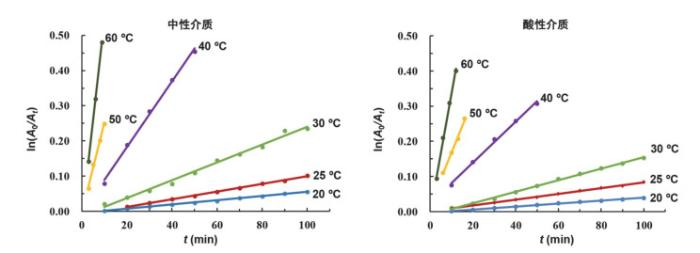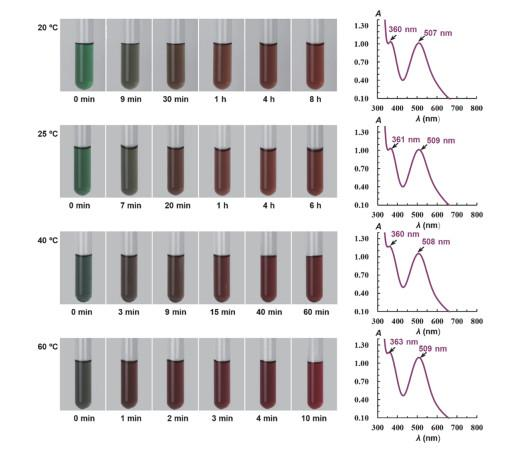## Laboratory Teaching Results and Discussions of "Determination of Kinetic Constants of the Hydrolysis Reaction of trans/cis-[Co(en)2Cl2]Cl Complexes"

Zhang Chunyan, Ouyang Xiaoqing, Ruan Chanzi, Lü Yinyun, Pan Rui, Weng Yuhua, Dong Zhiqiang, Xu Zhenling, Peng Shunü, Li Huamin, Ren Yanping,

 基金资助: 教育部2020年产学合作协同育人项目.  2020020600292021年度教育部“基础学科拔尖学生培养计划2.0”研究课题.  20212058Abstract

This paper mainly introduces the laboratory teaching implementation results and discussions of "determination of kinetic constants of the hydrolysis reaction of trans/cis-[Co(en)2Cl2]Cl complexes", which has been carried out for 10 years and particularly designed for top-talented chemistry-majored students at Xiamen University. Students were guided to discuss and explore the effects of reaction medium and temperature on the hydrolysis reaction of trans/cis-[Co(en)2Cl2]Cl, based on the dynamic experimental data and results. In light of this, students could directly understand the differences in the hydrolysis reaction kinetics behavior of trans/cis isomer with different structure, and the meaning of "structure decides the nature". Their ability of induction, summary, analysis, judgment and critical thinking can be cultivated.

Keywords： trans/cis-[Co(en)2Cl2]Cl ; Kinetics of hydrolysis reaction ; Analysis and discussion of experimental results

Zhang Chunyan. Laboratory Teaching Results and Discussions of "Determination of Kinetic Constants of the Hydrolysis Reaction of trans/cis-[Co(en)2Cl2]Cl Complexes". University Chemistry[J], 2021, 36(8): 2011035-0 doi:10.3866/PKU.DXHX202011035

### 图1### 1 trans-[Co(en)2Cl2]Cl配合物在中性和酸性介质中水解反应动力学实验结果及分析探讨

trans-[Co(en)2Cl2]Cl配合物在中性和酸性介质中能够发生水解反应，即

$trans-{{\underset{绿色}{\mathop{\left[ \text{Co}{{\left( \text{en} \right)}_{\text{2}}}\text{C}{{\text{l}}_{\text{2}}} \right]}}\, }^{\text{+}}}+{{\text{H}}_{\text{2}}}\text{O}\xrightarrow{{{\text{H}}_{\text{2}}}\text{O}或{{\text{H}}^{+}}}cis/trans-{{\underset{红色混合物}{\mathop{\left[ \text{Co}{{\left( \text{en} \right)}_{\text{2}}}\left( {{\text{H}}_{\text{2}}}\text{O} \right)\text{Cl} \right]}}\, }^{\text{2+}}}+\text{C}{{\text{l}}^{-}}$

### 1.1 室温下trans-[Co(en)2Cl2]Cl配合物在中性和酸性介质中水解反应速率常数测定

 t/min 中性介质 酸性介质 t/min 中性介质 酸性介质 A ln(A0/At) A ln(A0/At) A ln(A0/At) A ln(A0/At) t0 = 0 0.600 0.570 t6 = 60 0.557 0.074 0.542 0.050 t1 = 10 0.589 0.019 0.565 0.009 t7 = 70 0.551 0.085 0.537 0.060 t2 = 20 0.581 0.032 0.559 0.019 t8 = 80 0.544 0.098 0.533 0.067 t3 = 30 0.575 0.042 0.556 0.025 t9 = 90 0.540 0.105 0.530 0.073 t4 = 40 0.569 0.053 0.551 0.034 t10 = 100 0.532 0.120 0.524 0.084 t5 = 50 0.564 0.062 0.547 0.041

### 图2### 图3### 图4### 图5T/K 中性介质 酸性介质 拟合方程 R2 k/min−1 lnk 拟合方程 R2 k/min−1 lnk 293 y = 6.0 × 10−4x − 0.0047 0.9967 6.0 × 10−4 −7.42 y = 4.2 × 10−4x − 0.0023 0.9960 4.2 × 10−4 −7.78 298 y = 1.1 × 10−3x − 0.0091 0.9978 1.1 × 10−3 −6.81 y = 8.2 × 10−4x + 0.0013 0.9977 8.2 × 10−4 −7.11 303 y = 2.5 × 10−3x − 0.0129 0.9902 2.5 × 10−3 −5.99 y = 1.6 × 10−3x − 0.0085 0.9981 1.6 × 10−3 −6.44 313 y = 9.3 × 10−3x − 0.0040 0.9966 9.3 × 10−3 −4.68 y = 5.8 × 10−3x + 0.0228 0.9953 5.8 × 10−3 −5.15 323 y = 2.6 × 10−2x − 0.0048 0.9922 2.6 × 10−2 −3.65 y = 1.5 × 10−2x + 0.0173 0.9930 1.5 × 10−2 −4.20 333 y = 5.6 × 10−2x − 0.0241 0.9992 5.6 × 10−2 −2.88 y = 3.4 × 10−2x − 0.0012 0.9970 3.4 × 10−2 −3.38

### 图6### 图7### 图8### 图9### 图10## 参考文献 原文顺序 文献年度倒序 文中引用次数倒序 被引期刊影响因子

Aline O. M. ; Paolla C. M. ; Lucas B. F. R. C. ; Lucas B. B. ; Jonas P. ; Alexandre G. S. P. Quim. Nova 2006, 29 (2), 385.

Preparatory Problems 43rd International Chemistry Olympiad 2011 Ankara, Turkey (第43届国际奥林匹克化学竞赛实验预备题, 土耳其, 2011年), Problem 31. [2020-12-19]. https://wenku.baidu.com/view/ed204423bcd126fff7050bb5.html

/

 〈〉